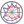Course title
Computational Models
 aiba akiraCourse content
As a computational model of functional programming languages, the lambda calculus is introduced in the class, mainly focused in its syntactic part. Besides, a mechanism to evaluate lambda terms called SECD machine, and another computational model for functional programming languages called combinatory logic are also described.
Purpose of class
Understaiding a computational model for functional programming langhuages by learning and understaiding the theory of lambda calculus.
Goals and objectives
1. Understanding theoretical aspects of programming languages.
2. Understanding a mathematical theory Lambda Calculus for modeling programming languages.
3. Understanding ways of thinking programs mathematically.
Language
English
Class schedule

Class schedule HW assignments (Including preparation and review of the class.) Amount of Time Required
1． Models of Programmina Languages (1): Necessity of Computational Models Understanding the contents of the class. 120minutes
2． Models of Programmina Languages (2): Lambda terms and some notions Understanding the contents of the class. 200minutes
3． Models of Programmina Languages (3): Beta reduction Understanding the contents of the class. 200minutes
4． Models of Programmina Languages (4): Confluency of Reduction Understanding the contents of the class. 120minutes
5． Models of Programmina Languages (5): Models of Programming Languages based on the Lambda Calculus Understanding the contents of the class. 200minutes
6． Typed Lambda Calculus (1): Constants and Base Types, Definition of Typed Lambda Terms Understanding the contents of the class. 210minutes
7． Typed Lambda Calculus (2): Simple Typed Lambda Calculus and Its Definition Understanding the contents of the class. 210minutes
8． Typed Lambda Calculus (3): de Brujin index and bound variables Understanding the contents of the class. 210minutes
9． Typed Lambda Calculus (4): Denotational Semantics of the Simple Typed Lambda Calculus, Set Theoretic Model Understanding the contents of the class. 210minutes
10． Typed Lambda Calculus (5): Denotational Semantics of the Simple Typed Lambda Calculus, Domain Theoretic Model Understanding the contents of the class. 210minutes
11． Typed Lambda Calculus (6): Axiomatic Semantics of the Simple Typed Lambda Calculus Understanding the contents of the class. 210minutes
12． Typed Lambda Calculus (7): What is Soundness and Completeness of the Axiomatic Semantics Understanding the contents of the class. 210minutes
13． Typed Lambda Calculus (8): Soundness of the Axiomatic Semantics Understanding the contents of the class. 210minutes
14． Typed Lambda Calculus (9): Completeness of the Axiomatic Semantics Understanding the contents of the class. 210minutes
Total. - - 2730minutes
Relationship between 'Goals and Objectives' and 'Course Outcomes'

Report Total.
1. 40% 40%
2. 40% 40%
3. 20% 20%
Total. 100% -
Evaluation method and criteria
Based on reports.
Students who can calculate beta-reductions, and understanding the basics of the lambda calculus will be graded as 60%.
Textbooks and reference materials
[Reference]
- H. P. Barendregt, "The Lambda Calculus - Its Syntex and Semantics", Studies in Logic and the Foundations of Mathematics, vol.103, Elsevier.
Prerequisites
Students must have experiences on programmin, and familiar with mathematical concepts like sets, functions.
Office hours and How to contact professors for questions
• Tuesday 12:30-13:00
Regionally-oriented
Non-regionally-oriented course
Development of social and professional independence
• Non-social and professional independence development course
Active-learning course
N/A
Course by professor with work experience
Work experience Work experience and relevance to the course content if applicatable
N/A N/A
Education related SDGs:the Sustainable Development Goals• 4.QUALITY EDUCATION